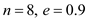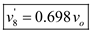# Three steel spheres of equal mass are suspended from the ceiling by cords of equal length which are spaced at a distance slightly greater than the diameter of the spheres. After being pulled back and released, sphere A hits sphere B, which then hits sphere C. Denoting by e the coefficient of institution between the spheres and by v0 the velocity of A just before it hits B, determine (a) the velocities of A and B immediately after the first collision (b) the velocities of B and C immediately after the second collision, (c) Assuming now that n spheres are suspended from the ceiling and that the first sphere is pulled back and released as described above, determine the velocity of the last sphere after it is hit for the first time. (d) Use the result of part c to obtain the velocity of the last sphere when n = 8 and e = 0.9.

Question-AnswerCategory: Engineering MechanicsThree steel spheres of equal mass are suspended from the ceiling by cords of equal length which are spaced at a distance slightly greater than the diameter of the spheres. After being pulled back and released, sphere A hits sphere B, which then hits sphere C. Denoting by e the coefficient of institution between the spheres and by v0 the velocity of A just before it hits B, determine (a) the velocities of A and B immediately after the first collision (b) the velocities of B and C immediately after the second collision, (c) Assuming now that n spheres are suspended from the ceiling and that the first sphere is pulled back and released as described above, determine the velocity of the last sphere after it is hit for the first time. (d) Use the result of part c to obtain the velocity of the last sphere when n = 8 and e = 0.9.

Three steel spheres of equal mass are suspended from the ceiling by cords of equal length which are spaced at a distance slightly greater than the diameter of the spheres. After being pulled back and released, sphere A hits sphere B, which then hits sphere C. Denoting by e the coefficient of institution between the spheres and by v0 the velocity of A just before it hits B, determine (a) the velocities of A and B immediately after the first collision (b) the velocities of B and C immediately after the second collision, (c) Assuming now that n spheres are suspended from the ceiling and that the first sphere is pulled back and released as described above, determine the velocity of the last sphere after it is hit for the first time. (d) Use the result of part c to obtain the velocity of the last sphere when n = 8 and e = 0.9.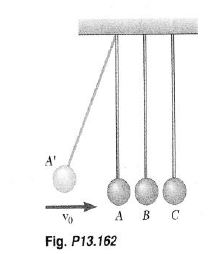Step: 1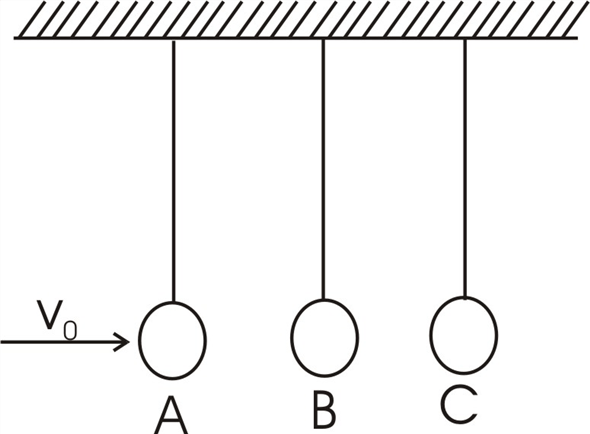Step: 2

Mass of the spheres,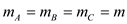Initial velocity of ‘A’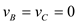Step: 3

(a) Velocity of A and B immediately after first collision
Writing conservation of momentum principle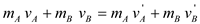OrOr…………….. (1)

Step: 4

AndOr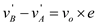…………… (2)

Step: 5

From equation (1),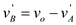Substituting this in equation (2),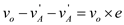OrStep: 6

From equation (2),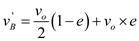Step: 7

(b) Velocity of B and C after the 2nd collision
Writing conservation of momentum principle,OrOr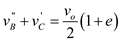………………. (3)

Step: 8

And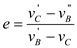Or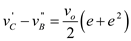…………….. (4)

Step: 9

From equation (3),Putting this in equation (4),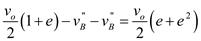Or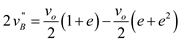OrStep: 10

From equation (4),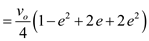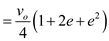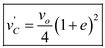Step: 11

(c) Put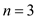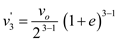Forballscollision,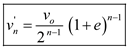Step: 12

(d) For